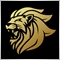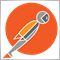# Can I create an OBJ_FIBO without the 23.6% level?503

Hi guys,

is it somehow possible to change the default creation of an OBJ_FIBO with only 38/50/61 levels? I tried to delete one level by using OBJPROP_LEVELS but this value is always zero after creating an OBJ_FIBO.

Any ideas? Maybe I can change the original code from Metaquotes in the library because I never use the 23.6 fibo level?Moderator
12670

Yes just (re)-code as indicator and edit out the unwanted parts.

If you don't know how you can talk to someone over here.

If you want to give it a try yourself you can see Fibonacci over here503

Marco vd Heijden:

Yes just (re)-code as indicator and edit out the unwanted parts.

If you don't know how you can talk to someone over here.

If you want to give it a try yourself you can see Fibonacci over here

Thank you, I know it's pretty easy to code it but I was wondering if I could use the built-in-Fibos and just modify the levels easily. Obviously it's not possible.Moderator
12670

Obviously not.Moderator
20339

It is not clear if you are asking about modifying a fibo by code or the object properties when placing the fibo on the chart manually.

By code you will find example in the documentation.

If adding a fibo to the chart manually, delete the level that you don't want, then every fibo that you add afterwards will not show that level.503

Keith Watford:

It is not clear if you are asking about modifying a fibo by code or the object properties when placing the fibo on the chart manually.

By code you will find example in the documentation.

If adding a fibo to the chart manually, delete the level that you don't want, then every fibo that you add afterwards will not show that level.

Sorry, I thought it was clear because I used the term OBJ_FIBO.

I wanted to create a fibo retracement by ObjectCreate() and wanted to delete the 23.6 level because it always appears when using ObjectCreate(). But in the meantime I made a small tool which calculates the three main fibo levels and draws three lines on the chart.Moderator
20339

Marbo:

Sorry, I thought it was clear because I used the term OBJ_FIBO.

Then you posted

Marbo:

Thank you, I know it's pretty easy to code it but I was wondering if I could use the built-in-Fibos and just modify the levels easily. Obviously it's not possible.

That's why I wasn't sure and my answer was using the built-in Fibo from the toolbar.Moderator
20339

I've thrown this together.

```//+------------------------------------------------------------------+
//|                                            Fibonacci Example.mq4 |
//|                                                    Keith Watford |
//|                                                                  |
//+------------------------------------------------------------------+
#property strict
#property indicator_chart_window
//+------------------------------------------------------------------+
struct val
{
double            LevelDouble;
string            LevelString;
string            Text;
color             Col;
ENUM_LINE_STYLE   Style;
};
//+------------------------------------------------------------------+
//--- input parameters
input string          FiboName      ="Fibonacci";            // Fibonacci Name
input string          InpLevels     ="0.38,0.50,0.61";       // Levels, separated by commas
input color           InpLevelColor =clrDodgerBlue;          // Fibonacci Line color
input ENUM_LINE_STYLE InpStyle      =STYLE_DASHDOTDOT;       // Style of lines
input int             InpWidth      =1;                      // Width of the lines //Note width only applies to solid lines

val    FibValues[];
int    Size;
//+------------------------------------------------------------------+
//| Custom indicator initialization function                         |
//+------------------------------------------------------------------+
int OnInit()
{
string tempArray[];
Size=StringSplit(InpLevels,',',tempArray);
ArrayResize(FibValues,Size);
for(int x=0;x<Size;x++)
{
FibValues[x].Col=InpLevelColor;
FibValues[x].Style=InpStyle;
FibValues[x].LevelDouble=StrToDouble(tempArray[x]);
FibValues[x].LevelString=DoubleToStr(FibValues[x].LevelDouble,3);
FibValues[x].Text=FibValues[x].LevelString+" ";
}
FiboLevelsSet(0,FiboName);
//---
return(INIT_SUCCEEDED);
}
//+------------------------------------------------------------------+
//| Custom indicator iteration function                              |
//+------------------------------------------------------------------+
int OnCalculate(const int rates_total,
const int prev_calculated,
const datetime &time[],
const double &open[],
const double &high[],
const double &low[],
const double &close[],
const long &tick_volume[],
const long &volume[],
{
//---

//--- return value of prev_calculated for next call
return(rates_total);
}
//+------------------------------------------------------------------+
//| Set number of levels and their parameters                        |
//+------------------------------------------------------------------+
bool FiboLevelsSet(const long      chart_ID=0,           // chart's ID
const string    name="Fibo")          // object name
{
//--- set the number of levels
ObjectSetInteger(chart_ID,name,OBJPROP_LEVELS,Size);
//--- set the properties of levels in the loop
for(int i=0;i<Size;i++)
{
//--- level value
ObjectSetDouble(chart_ID,name,OBJPROP_LEVELVALUE,i,FibValues[i].LevelDouble);
//--- level color
ObjectSetInteger(chart_ID,name,OBJPROP_LEVELCOLOR,i,FibValues[i].Col);
//--- level style
ObjectSetInteger(chart_ID,name,OBJPROP_LEVELSTYLE,i,FibValues[i].Style);
//--- level width
ObjectSetInteger(chart_ID,name,OBJPROP_LEVELWIDTH,i,InpWidth);
//--- level description
ObjectSetString(chart_ID,name,OBJPROP_LEVELTEXT,i,FibValues[i].Text+"%\$");
}
//--- successful execution
return(true);
}
//+------------------------------------------------------------------+ ```

This is as an indicator, but you may prefer it as a Script or whatever. Anyway, it should give you some ideas and I am sure that you will be able to integrate some of it into your code.

You will need to draw a standard Fibo on your chart and then put the name of that fibo in the input, then the fibo should be redrawn with the new levels when the indicator is re-initialized.

I hope that it helps.2392

And you could also use the Fibo class...

```#include <chartobjects/chartobjectsfibo.mqh>
class MyFibo : public CChartObjectFibo {
public: bool create(const double &levels[]) {
bool res = true;
res &= this.Create(0, "MyFibo", 0, 0, 0.0, 0, 0.0);
int total = ArraySize(levels);
res &= this.LevelsCount(total);
res &= this.RayRight(true);
for(int i=0; i<total; i++) {
res &= this.LevelValue(i, levels[i]);
res &= this.LevelDescription(i, string(levels[i]));
}
return res;
}
};

MyFibo fibo;

int OnInit()
{
double levels[] = {0.382, 0.5, 0.618};
if(!fibo.create(levels))
return INIT_FAILED;
return INIT_SUCCEEDED;
}

void OnTick()
{
int i1 = iLowest(_Symbol, _Period, MODE_LOW, 20);
int i2 = iHighest(_Symbol, _Period, MODE_HIGH, 20);
fibo.Time(0, iTime(_Symbol, _Period, i1));
fibo.Price(0, iLow(_Symbol, _Period, i1));
fibo.Time(1, iTime(_Symbol, _Period, i2));
fibo.Price(1, iHigh(_Symbol, _Period, i2));
}
```